$$\def\loading{......LOADING......Please Wait} \def\RR{\bf R} \def\real{\mathbb{R}} \def\bold#1{\bf #1} \def\d{\mbox{Cord}} \def\hd{\widehat \mbox{Cord}} \DeclareMathOperator{\cov}{cov} \DeclareMathOperator{\var}{var} \DeclareMathOperator{\cor}{cor} \newcommand{\ac}{\left\{#1\right\}} \DeclareMathOperator{\Ex}{\mathbb{E}} \DeclareMathOperator{\diag}{diag} \newcommand{\bm}{\boldsymbol{#1}} \def\wait{......LOADING......Please Wait}$$

## Pathway Lasso: Estimate and Select Multiple Mediation Pathways

### Xi (Rossi) LUO

Brown University
Department of Biostatistics
Center for Statistical Sciences
Computation in Brain and Mind
Brown Institute for Brain Science
Brown Data Science Initiative
ABCD Research Group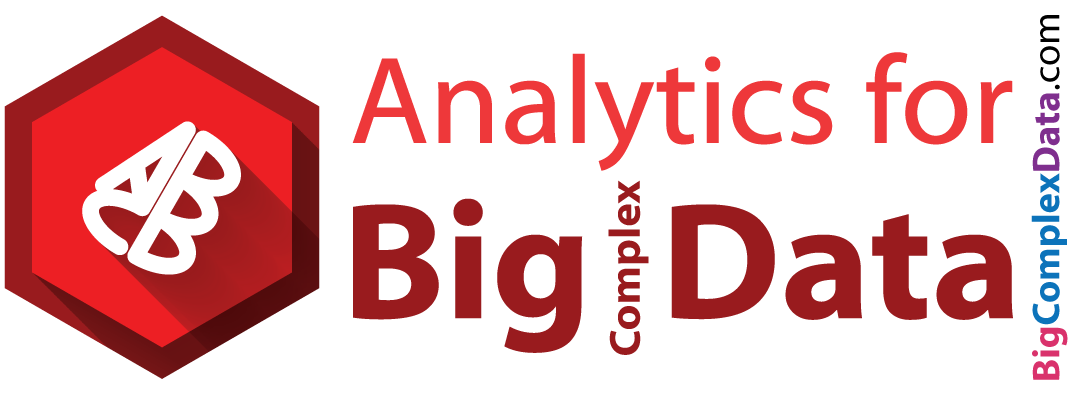IBC, Barcelona Spain
July 10, 2018

Funding: NIH R01EB022911, P20GM103645, P01AA019072, P30AI042853; NSF/DMS (BD2K) 1557467

## Co-AuthorYi Zhao
Currently postdoc at Johns Hopkins Biostat

# Slides viewable on web: bit.ly/ibc1807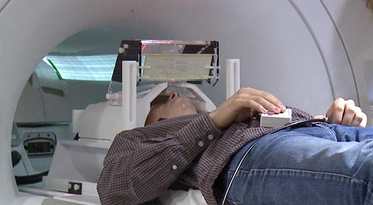• Story vs Math task: listen to story (treatment stimulus) or math questions (control), eye closed
• Not resting-state: "rest" in scanner

Goal: how brain processes story/math differently?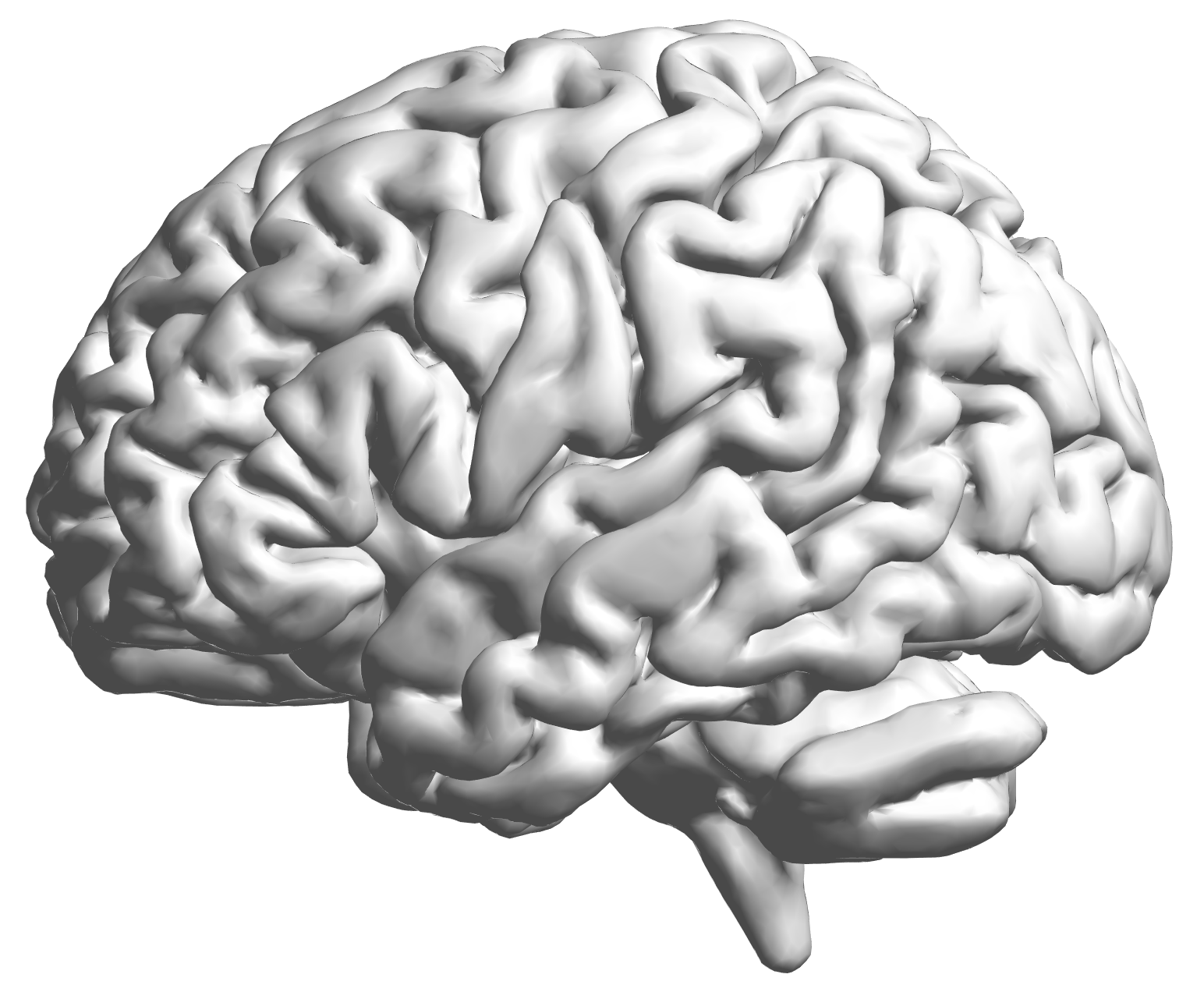fMRI data: blood-oxygen-level dependent (BOLD) signals from each cube/voxel (~millimeters), $10^5$ ~ $10^6$ voxels in total.

## Conceptual Model with Stimulus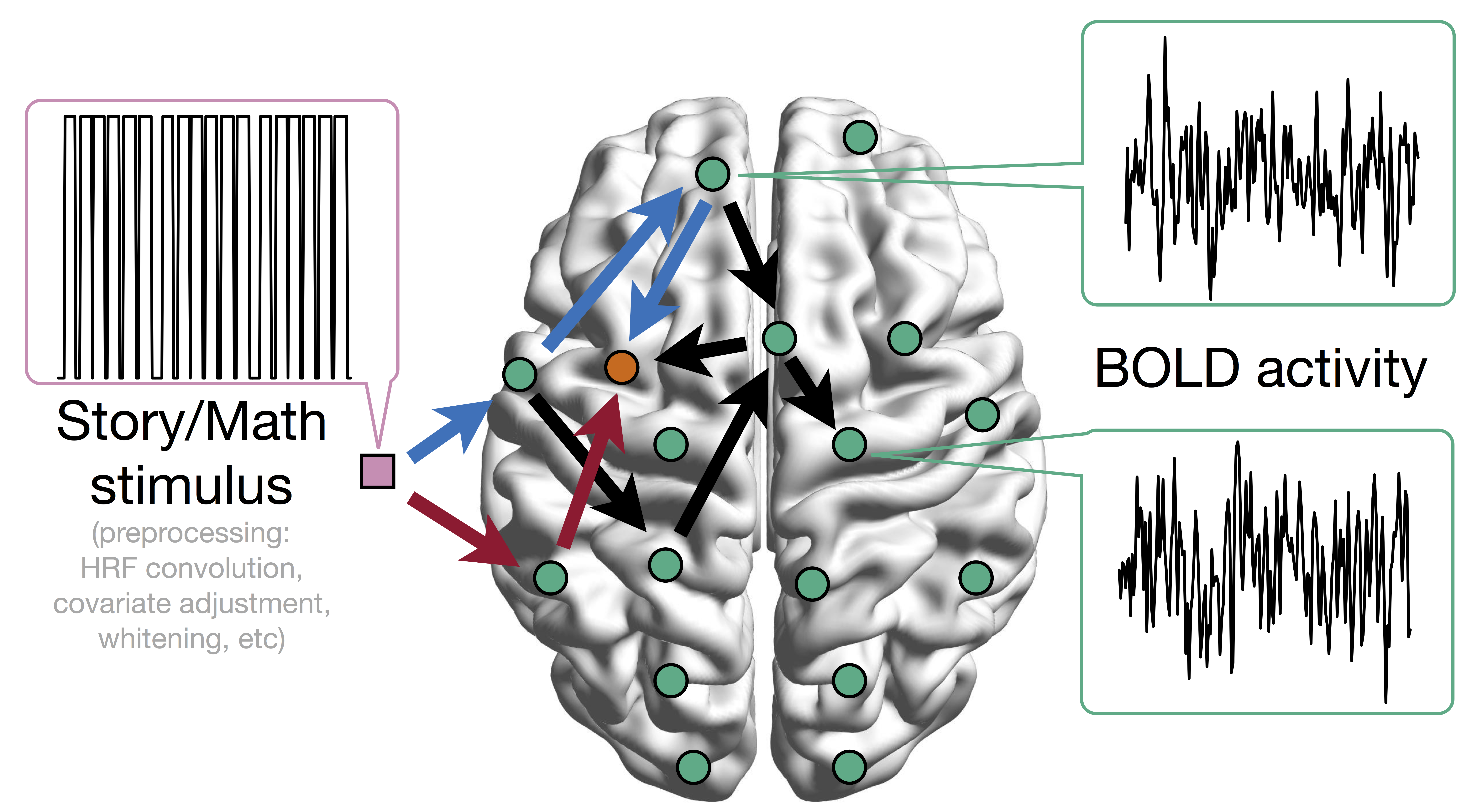Sci Goal: quantify red, blue, and other pathways
from stimulus to orange outcome region activityHeim et al, 09

## Other Potential Applications

• Genomics/genetics/proteomics
• Multiple genetic pathways
• Integrating multiple sources
• Imaging and genetics
• EHR
• Common theme: many potential pathways/mediators to disease outcomes

# Model

## Mediation Analysis and SEM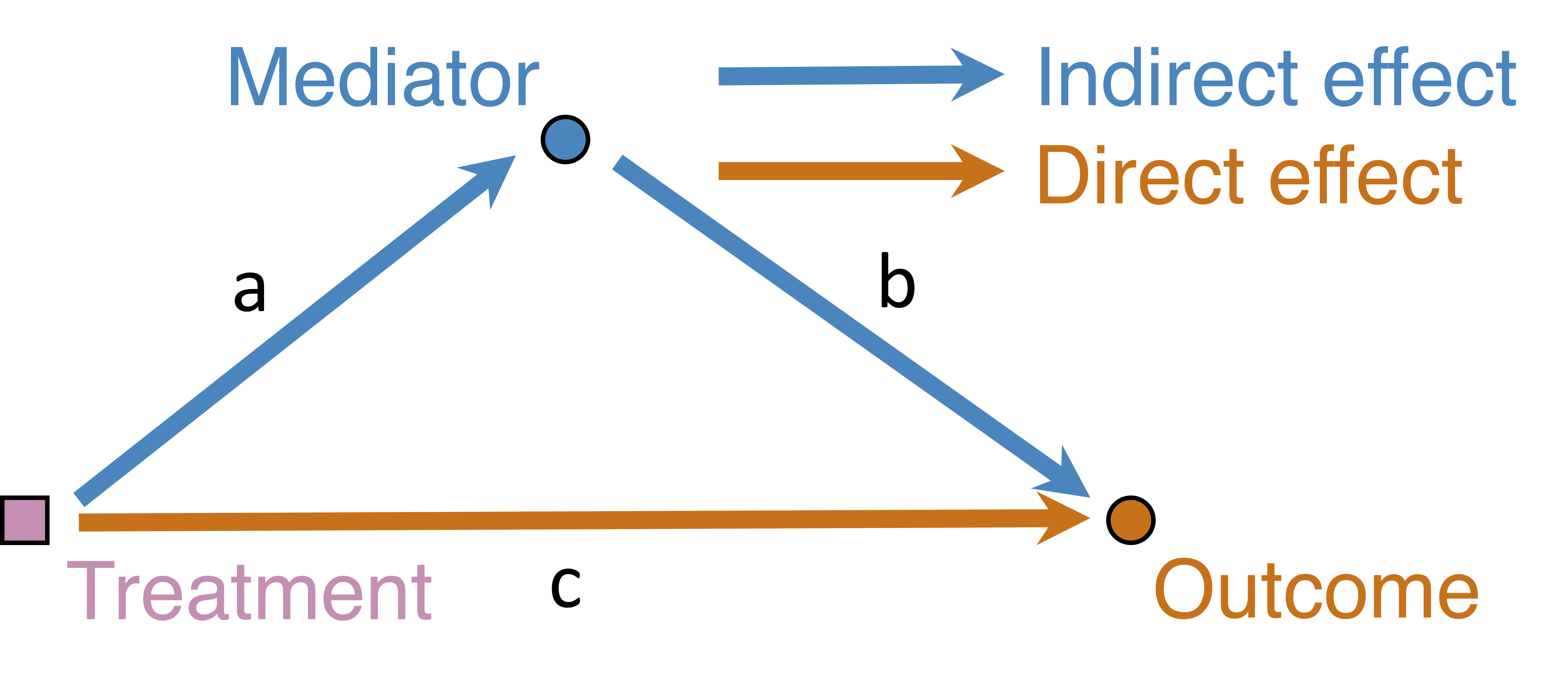\begin{align*}M = Z a + { \epsilon_1}, \qquad R = Z c + M b + { \epsilon_2}\end{align*}
• Indirect effect: $a \times b$; direct: $c$
• Mediation analysis
• Baron&Kenny, 86; Sobel, 82; Holland 88; Preacher&Hayes 08; Imai et al, 10; VanderWeele, 15;...

## Multiple (Full) Pathway Model Daniel et al, 14• Stimulus $Z$, $K$ mediating brain regions $M_1, \dotsc, M_K$, Outcome region $R$
• Strength of activation ($a_k$) and connectivity ($b_k$, $d_{ij}$)
• Potential outcomes too complex, e.g. $K = 2$ Daniel et al, 14

## Practical Considerations

• The previous model requires specifying the order of mediators, usually unknown in many experiments
• We don't know yet the order of brain regions
• fMRI: not enough temporal resolution to determine the order
• Theoretically and computationally challenging with a large number of mediators
• High dimensional (large $p$, small $n$) setting: $K>n$

## Mediation Analysis in fMRI

• Parametric Wager et al, 09 and functional Lindquist, 12 mediation, under (approx.) independent errors
• Stimulus $\rightarrow$ brain $\rightarrow$ user reported ratings, one mediator
• Usual assumption: $U=0$ and $\epsilon_1 \bot \epsilon_2$
• Parametric and multilevel mediation Yi and Luo, 15, with correlated errors for two brain regions
• Stimulus $\rightarrow$ brain region A $\rightarrow$ brain region B, one mediator
• Correlations between $\epsilon_1$ and $\epsilon_2$
• This talk: multiple mediator and multiple pathways
• High dimensional: more mediators than sample size
• Dimension reduction: optimization Chen et al, 15, testing Huang et al, 16

## Our Reduced Pathway Model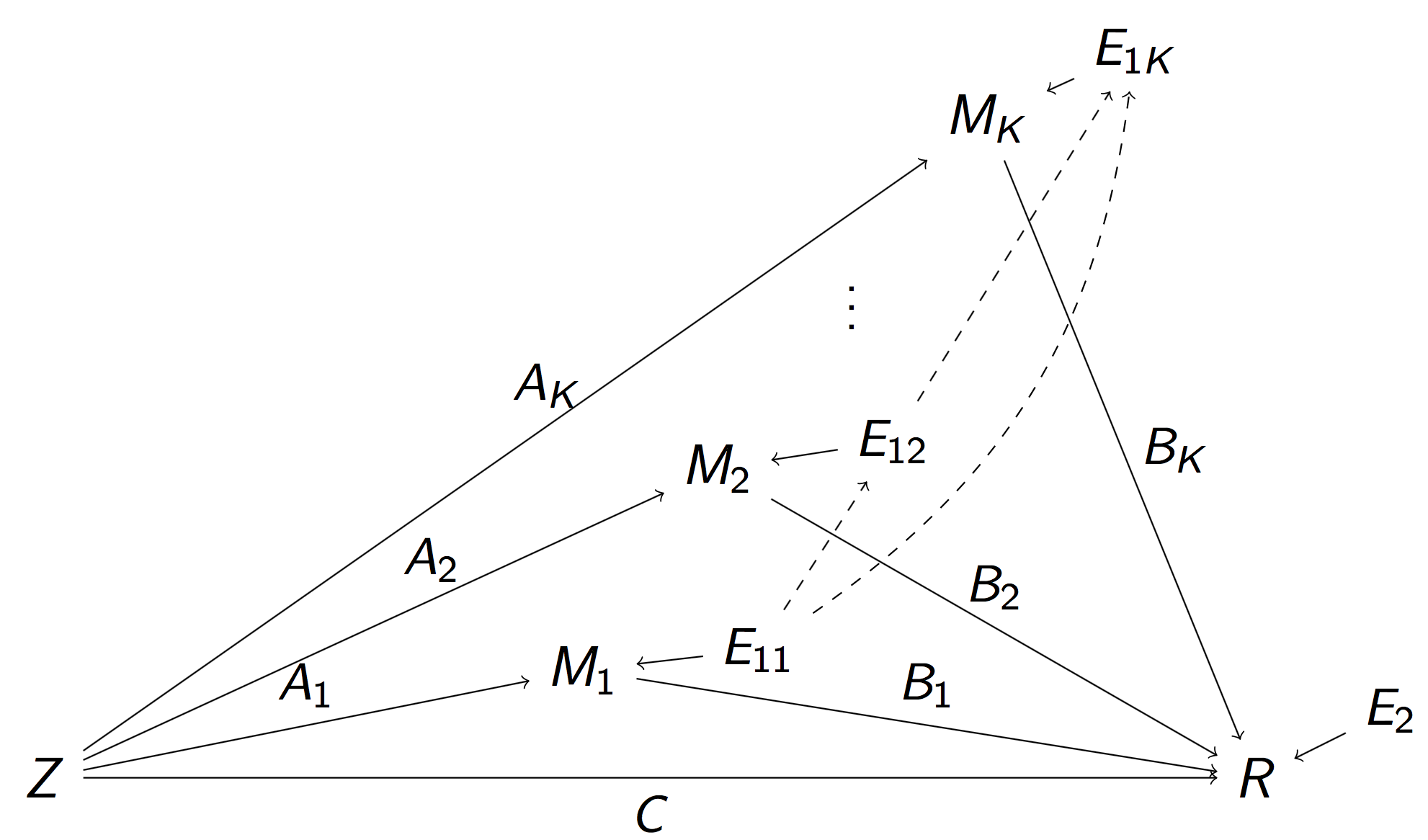\scriptsize \begin{align}M_k & = Z A_k + E_{1k},\, k=1,\dotsc, K\\ \scriptsize R & = Z C + \sum_{k=1}^{K} M_k B_k + E_2 \end{align}
• $A_k$: "total" effect of $Z$→$M_k$; $B_k$: $M_k$→R
• Pathway effect: $A_k \times B_k$; Direct: $C$

## Two ModelsFull ModelReduced Model

• Proposition: Our "total-effect" parameters are linearly related or equivalent to the "individual-effect" parameters in the full model
• $C=c$ and $B_k=b_k$, $k=1,\dotsc, K$, are the same in both models
• $A_k$ and $a_k$ in two models are linearly related
• $A_k \times B_k$ interpreted as the "total" effect when $M_k$ is the last mediator Imai & Yamamoto, 13

## Additional Relation to Full Model

• Proposition: Our $E_k$'s are correlated, but won't affect point estimation consistency (affect variance)
• The price of ignoring the order
• Related to causally independent mediators if assuming indepdent $E_k$
• Reduced model: a first step to select mediators
• Strong overall inflow/outflow of a mediator

## Causal Assumptions

• We impose standard causal mediation assumptions:
• SUTVA
• Model correctly specified
• Observed is one reliazation of the potential outcomes
• Randomized $Z$
• No unmeasured confounding/sequential ignorability
• Similar assumptions discussed in Imai & Yamamoto, 13; Daniel et al, 14; VanderWeele, 15
• Could be too strong or sensitivity analysis Imai & Yamamoto, 13

# Method

## Regularized Regression

• Minimize the penalized least squares criterion
$$\scriptsize \sum_{k=1}^K \| M_k - Z A_k \|_2^2 + \| R - Z C - \sum_k M_k B_k \|_2^2 + \mbox{Pen}(A, B)$$
The choice of penalty $\mbox{Pen}(\cdot)$ to be discussed
• All data are normalized (mean=0, sd=1)
• Want to select sparse pathways for high-dim $K$
• Alternative approach: two-stage LASSO Tibshirani, 96 to select sparse $A_k$ and $B_k$ separately: $$\scriptsize \sum_{k=1}^K \| M_k - Z A_k \|_2^2 + \lambda \sum_k | A_k | \\ \scriptsize \| R - Z C - \sum_k M_k B_k \|_2^2 + \lambda \sum_k |B_k|$$

## Penalty: Pathway LASSO

• Select strong pathways effects: $A_k \times B_k$
• TS-LASSO: shrink to zero when $A$&$B$ moderate but $A\times B$ large
• Penalty (prototype) $$\scriptsize \lambda \sum_{k=1}^K |A_k B_k|$$
• Non-convex in $A_k$ and $B_k$
• Computationally heavy and non-unique solutions
• Hard to prove theory
• We propose the following general class of penalties$$\scriptsize \lambda \sum_{k=1}^K ( |A_k B_k| + \phi A_k^2 + \phi B_k^2)$$
Theorem $$v(a,b) = |a b| + \phi (a^2 + b^2)$$ is convex if and only if $\phi\ge 1/2$. Strictly convex if $\phi > 1/2$.

Contour Plot of Different Penalties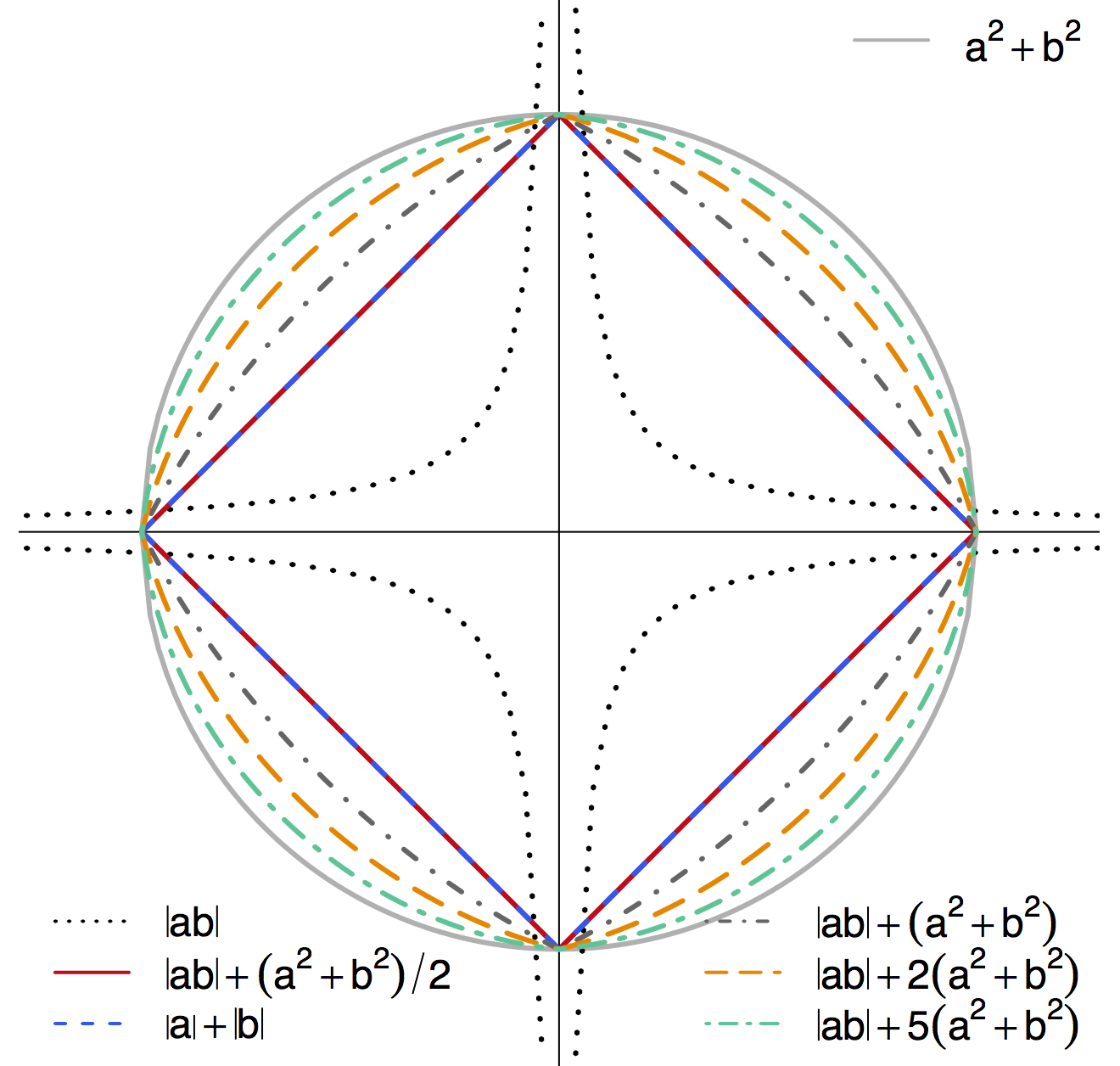• Non-differentiable at points when $a\times b = 0$
• Shrink $a\times b$ to zero
• Special cases: $\ell_1$ or $\ell_2$
• TS-LASSO: different $|ab|$ effects though $|a|+|b|$ same$|ab|$

Non-convex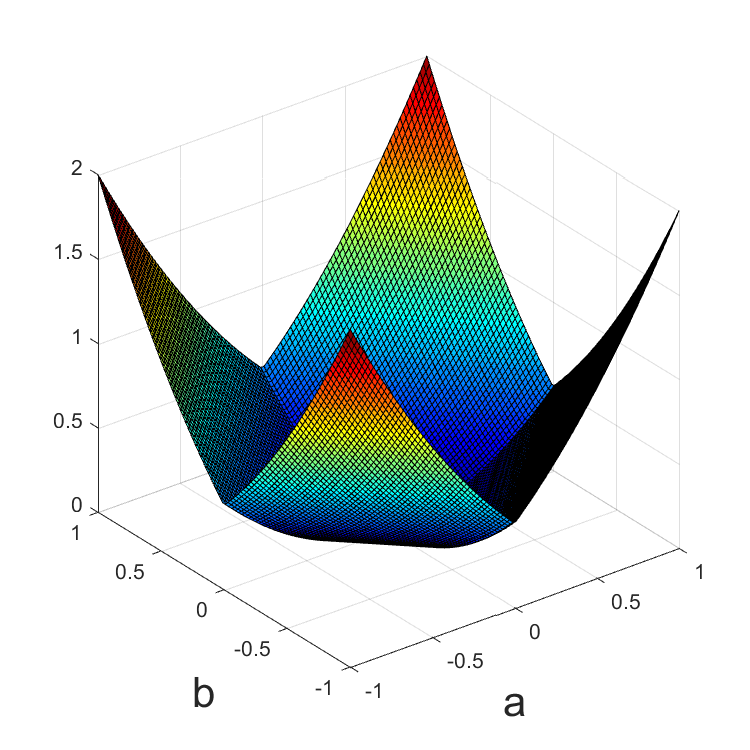$|ab|+\phi (a^2 + b^2)$

Pathway Lasso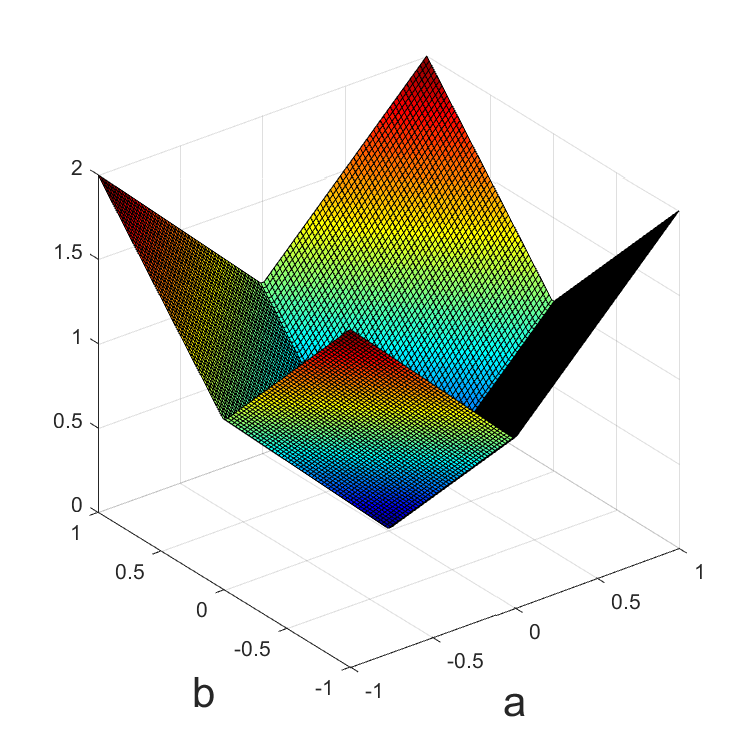$|a| + |b|$

Two-stage Lasso

Pathway Lasso is a family of (convex) penalties for products

• SEM/regression loss: $u$; Non-differnetiable penalty: $v$
• ADMM to address differentiability \begin{aligned} \text{minimize} \quad & u(\Theta,D)+v(\alpha,\beta) \\ \text{subject to} \quad & \Theta=\alpha, \\ & D=\beta, \\ & \Theta e_{1}=1, \end{aligned}
• Augmented Lagrangian for multiple constraints
• Iteratively update the parameters
• We derive theorem on explicit (not simple) updates

## Asymptotic Theory

Theorem: Under regularity conditions,
$$\Ex (Z \sum_k \hat{A}_k\hat{B}_k - Z \sum_k {A}^*_k {B}^*_k )^2 \le O(s \kappa \sigma n ^{-1/2} (\log K)^{1/2}),$$ where $s = \#\{j: B_j^* \ne 0\}$ and $\kappa =\max_j |B_j|$. With high probability,
$$\| \hat{A} \hat{B} - A^* B^* \| \le O(s \kappa \sigma n ^{-1/2} (\log K)^{1/2})$$

## Complications

• Mixed norm penalty $$\mbox{PathLasso} + \omega \sum_k (|A_k| + |B_k|)$$
• Tuning parameter selection by cross validation
• Reduce false positives via thresholding Johnston and Lu, 09
• Inference/CI: bootstrap after refitting
• Remove false positives with CIs covering zero Bunea et al, 10

# Simulations

## Simulations

• Our PathLasso compares with TSLasso
• Simulate with varying error correlations
• Tuning-free comparison: performance vs tuning parameter (estimated effect size)
• PathLasso outperforms under CV

## Pathway RecoveryROCF1 Score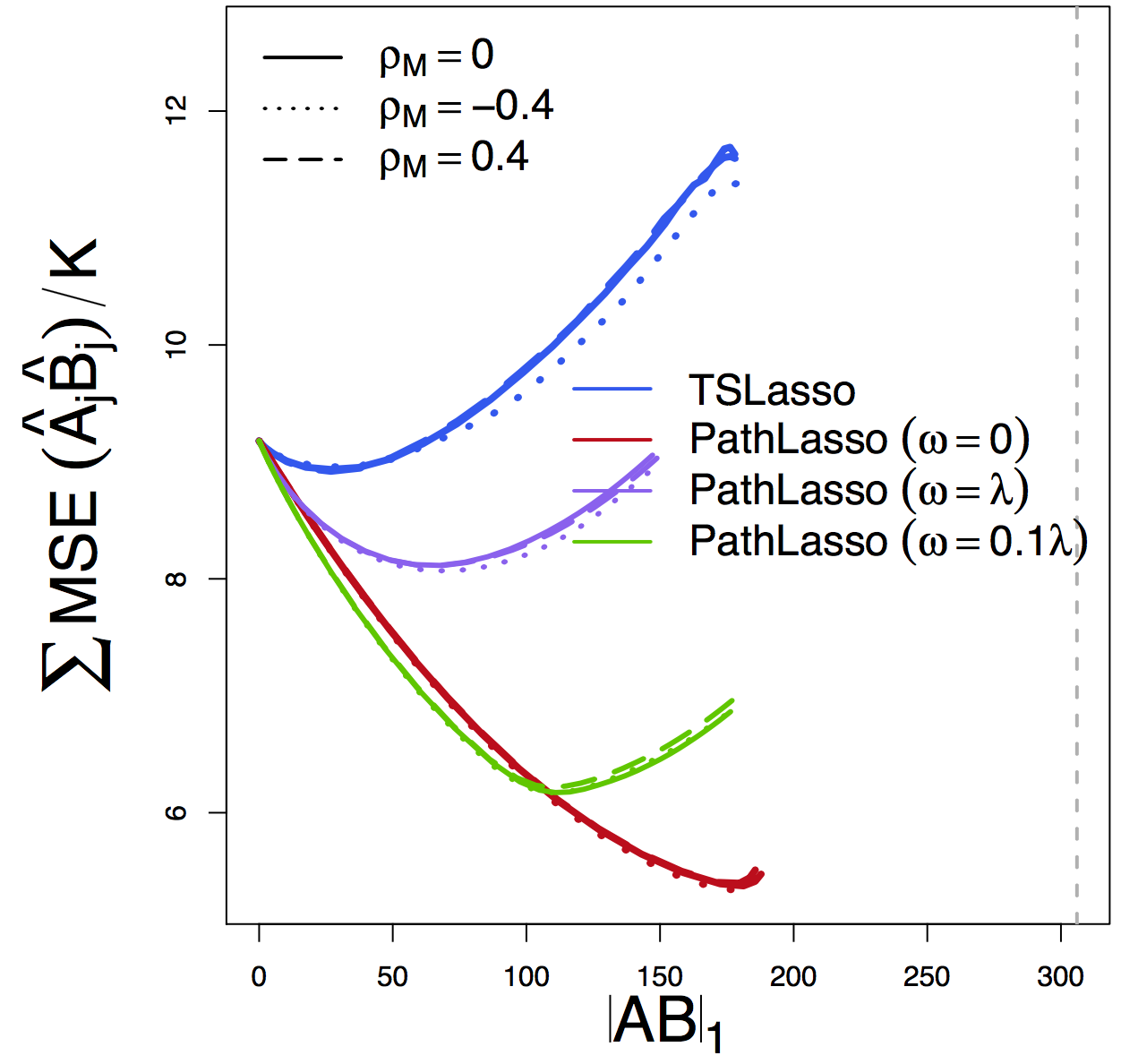MSE

Our PathLasso (red) outperforms two-stage Lasso (blue)

Other curves: variants of PathLasso and correlation settings

# Real Data: HCP

## Data: Human Connectome Project

• Two sessions (LR/RL), story/math task Binder et al, 11
• gICA reduces voxel dimensions to 76 brain maps
• ROIs/clusters after thresholding
• Apply to two sess separately, compare replicability
• Jaccard: whether selected pathways in two runs overlap
• $\ell_2$ diff: difference between estimated path effects
• Tuning-free comparisons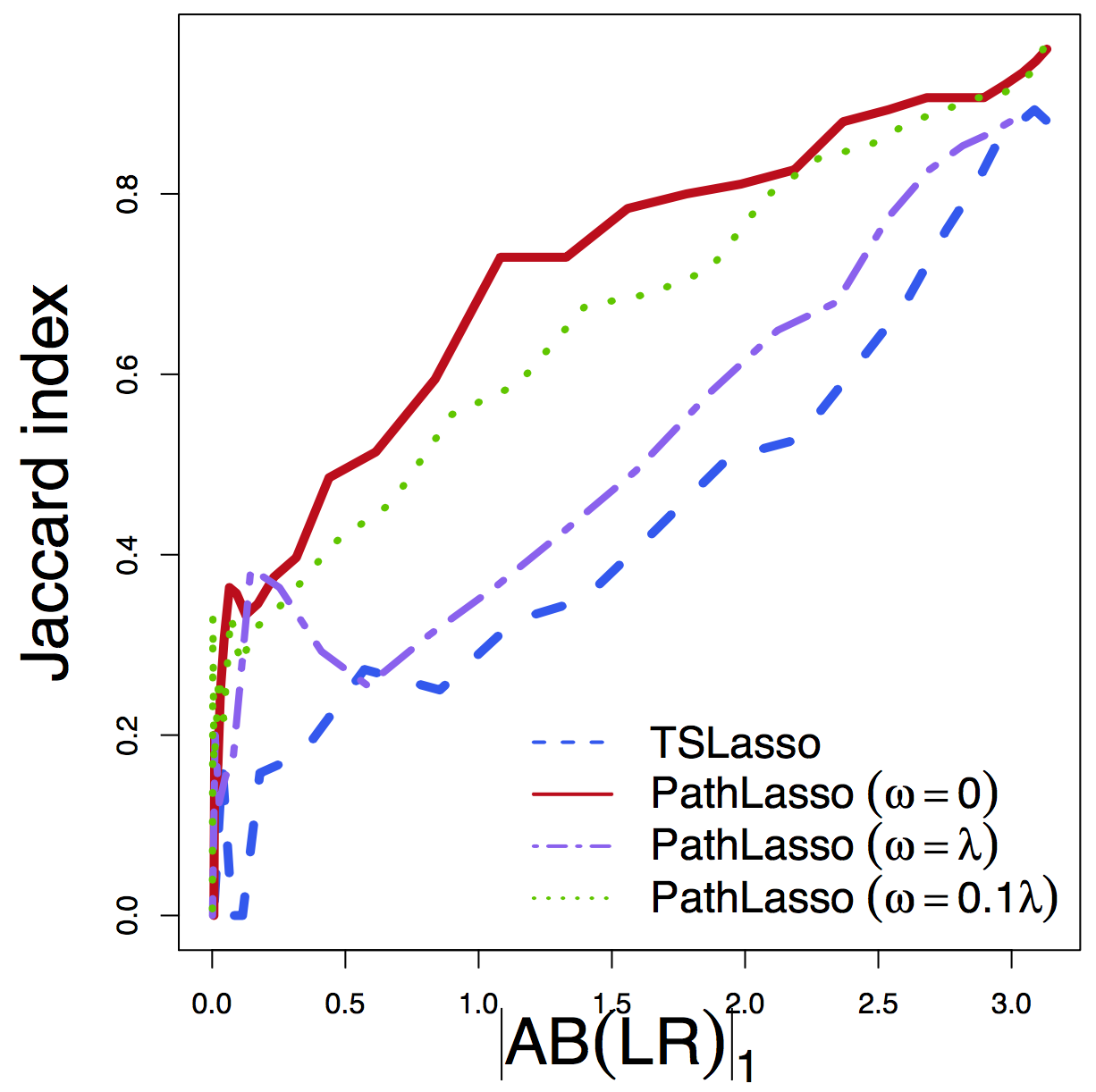Jaccard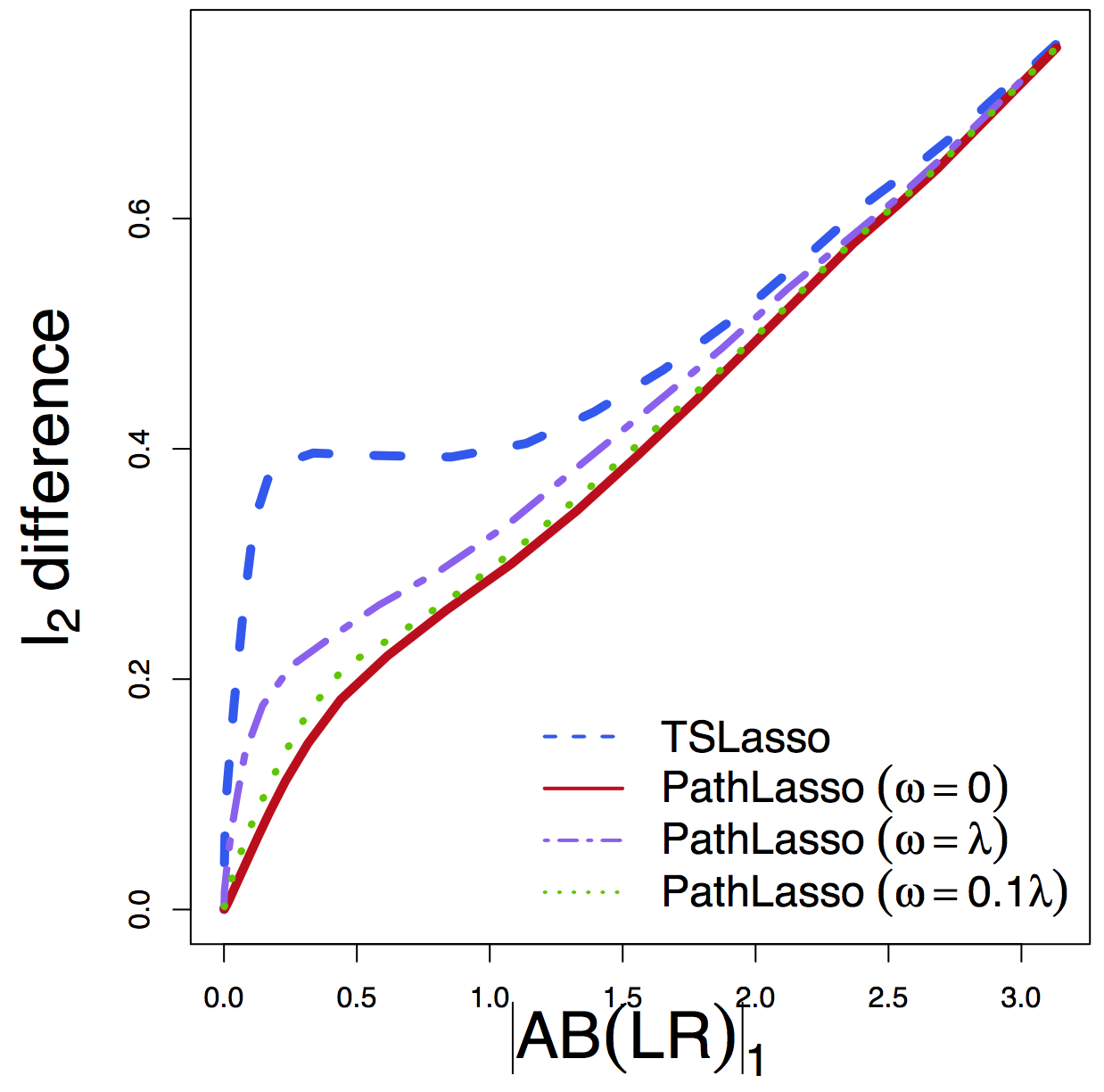$\ell_2$ Diff

Regardless of tuning, our PathLasso (red) has smaller replication diff (selection and estimation) than TSLasso (blue)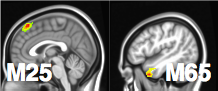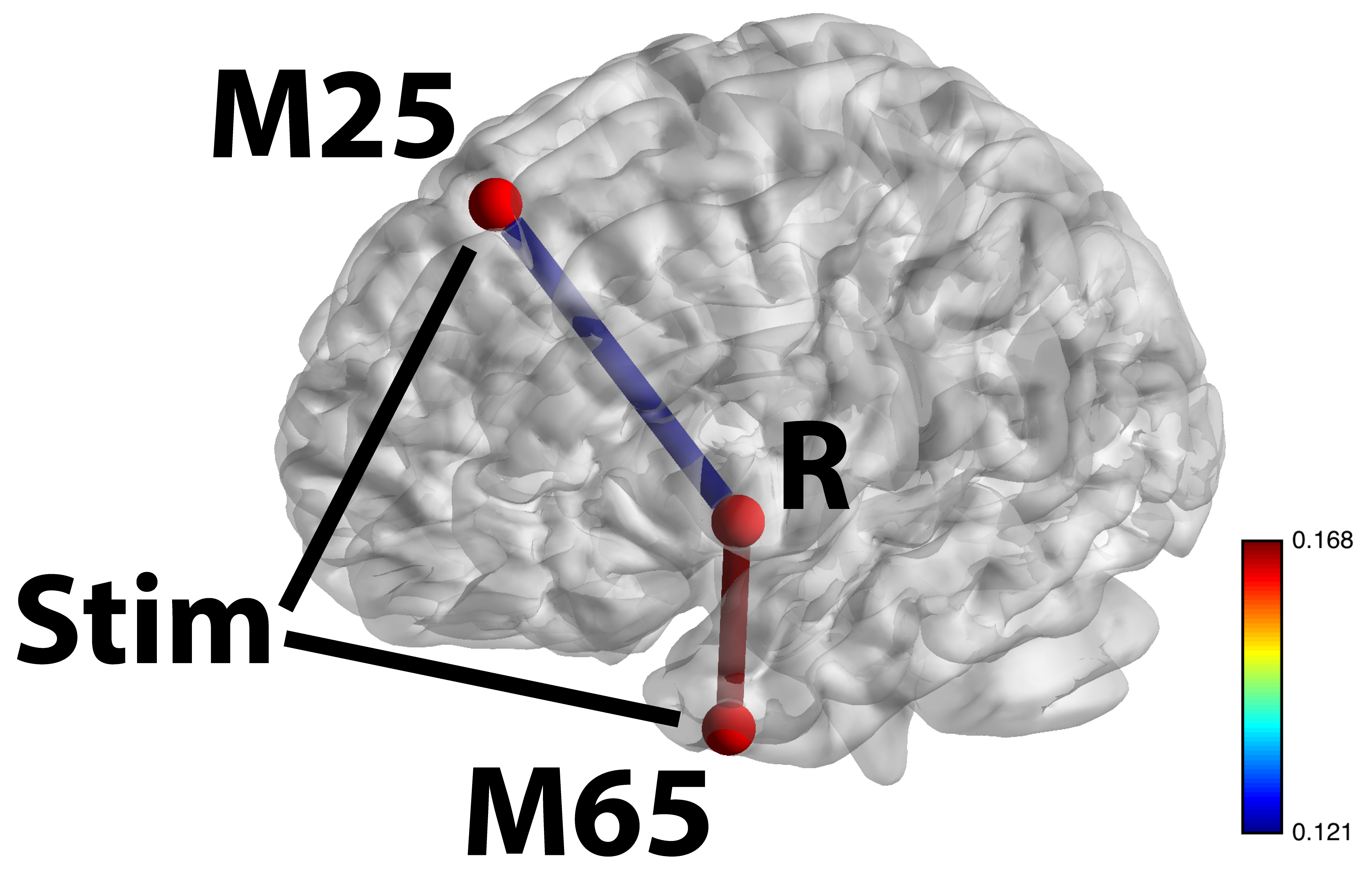Stim-M25-R and Stim-M65-R significant shown largest weight areas

• M65 responsible for language processing, larger flow under story
• M25 responsible for uncertainty, larger flow under math

## Summary

• High dimensional pathway model
• Penalized SEM for pathway selection and estimation
• Convex optimization for non-convex products
• Sufficient and necessary condition
• Algorithmic development for complex optimization
• Improved estimation and selection accuracy
• Higher replicability using HCP data
• Manuscript: Pathway Lasso (arXiv 1603.07749)
• Limitations: causal assumptions, covaraites, interactions, error correlations Rpkg: macc, time series gma, functional cfma